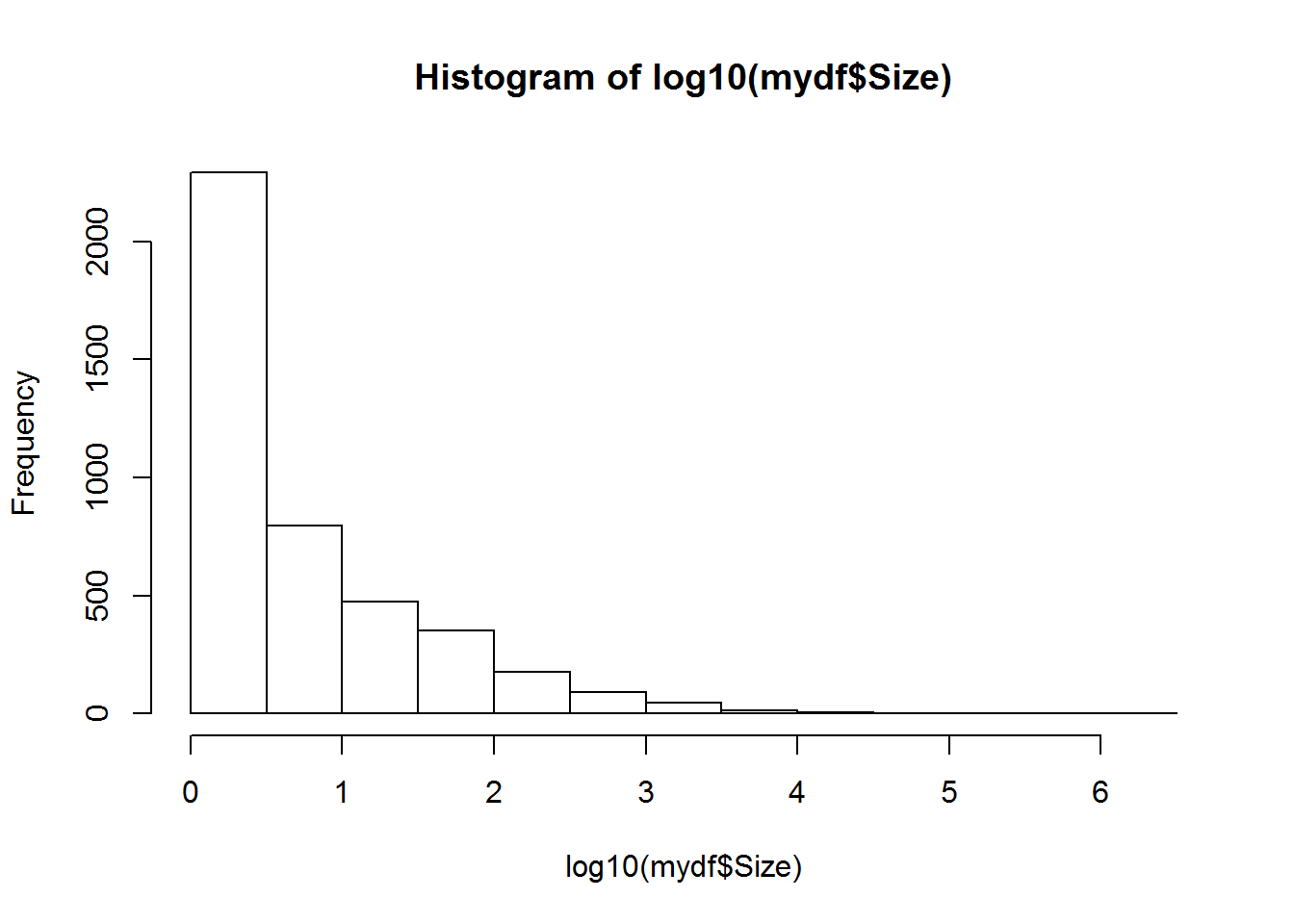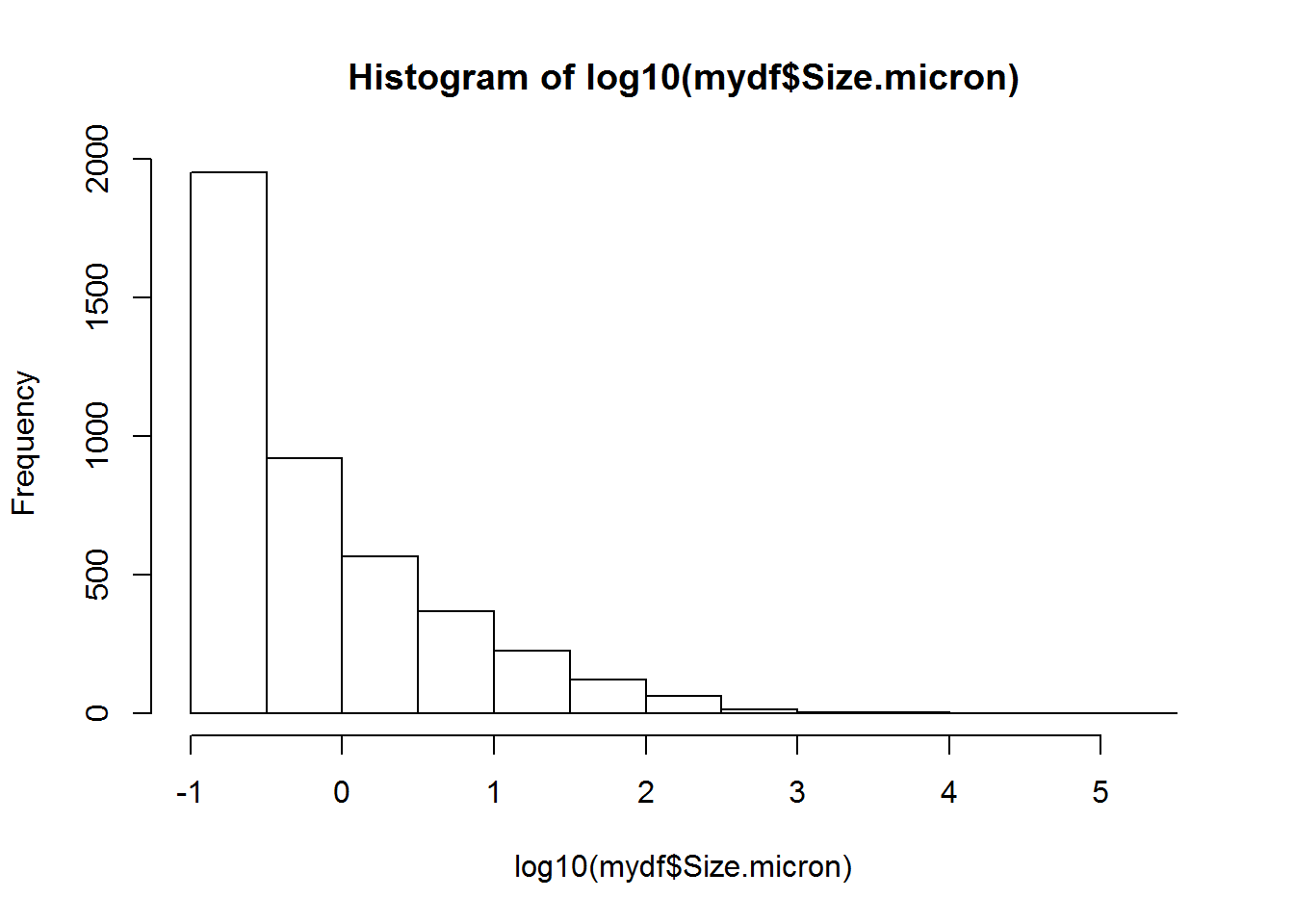# Finding clumps / aggregates / micro-colonies

This is a tutorial on how to locate clumps of pixels by joining adjacent pixels

library(RCon3D)

## Finding the images

path <- "/Data"
imgs <- findIMG(path)

## Detect clumps

Set kern.smooth to smooth the images prior to finding aggregates (Recommended).

agg <- clumps(imgs, channels = "xan", kern.smooth = c(3,3,3), zstep = 0.25, pwidth = 0.75)
##
|
|                                                                 |   0%

## Plot clumps

Giving the function zstep and pwidth in microns draws the plot to scale.

Here we set a threshold of 100 cubic microns. Clumps smaller than this are not plotted. If thresh.m = FALSE it would set the threshold to 100 pixels instead.

By default the clumps are coloured by their clump ID. Colours are recycled.

Note that the plot is an interactive 3D plot, and is therefore not visible in this tutorial.

clumps_plot(agg, zstep = 0.25, pwidth = 0.75, thresh = 100, thresh.m = TRUE)

## Size of clumps

agg[] contains a data.frame with information on each aggregate/clump

mydf <- agg[]
hist(log10(mydf$Size))Because we gave the zstep and pwidth to the clumps function we also get the size in cubic microns: hist(log10(mydf$Size.micron))